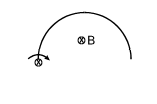Motional EMF
Question

# A semicircular wire of radius R is rotated with constant angular velocity $\mathrm{\omega }$ about an axis passing through one end and perpendicular to the plane of the wire. There is a uniform magnetic field of strength B. The induced e.m.f. between the ends is:Moderate
Solution

## $\begin{array}{l}\mathrm{e}=\frac{1}{2}\mathrm{B}\left({\mathrm{l}}^{2}\right)\mathrm{\omega }\\ =\frac{1}{2}\mathrm{B}{\left(2\mathrm{r}\right)}^{2}\mathrm{\omega }\\ =2{\mathrm{Br}}^{2}\mathrm{\omega }\end{array}$

Get Instant Solutions